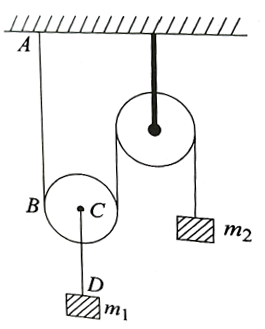Travelling wave
Question

# Two pulleys of negligible mass are connected to two blocks of mass m1 and m2 by two strings of the same material and the same cross-sectional area as shown in Fig. The speed of a transverse wave in string AB is v1 and that in string CD is v2. The ratio $\frac{{\mathrm{v}}_{1}}{{\mathrm{v}}_{2}}$isModerate
Solution

## Speed of transverse wave in a stretched string is $\mathrm{v}=\sqrt{\frac{\mathrm{T}}{\mathrm{\mu }}}$ where T is the tension in the string and $\mathrm{\mu }$ is the mass per unit length of the string. Since strings AB and CD have the same density and the same cross-sectional area, they have the same $\mathrm{\mu }$. Hence $\mathrm{v}\propto \sqrt{\mathrm{T}}$If the tension in CD is T, the tension in string AB is $\frac{\mathrm{T}}{2}.$So the correct choice is (d).

Get Instant Solutions